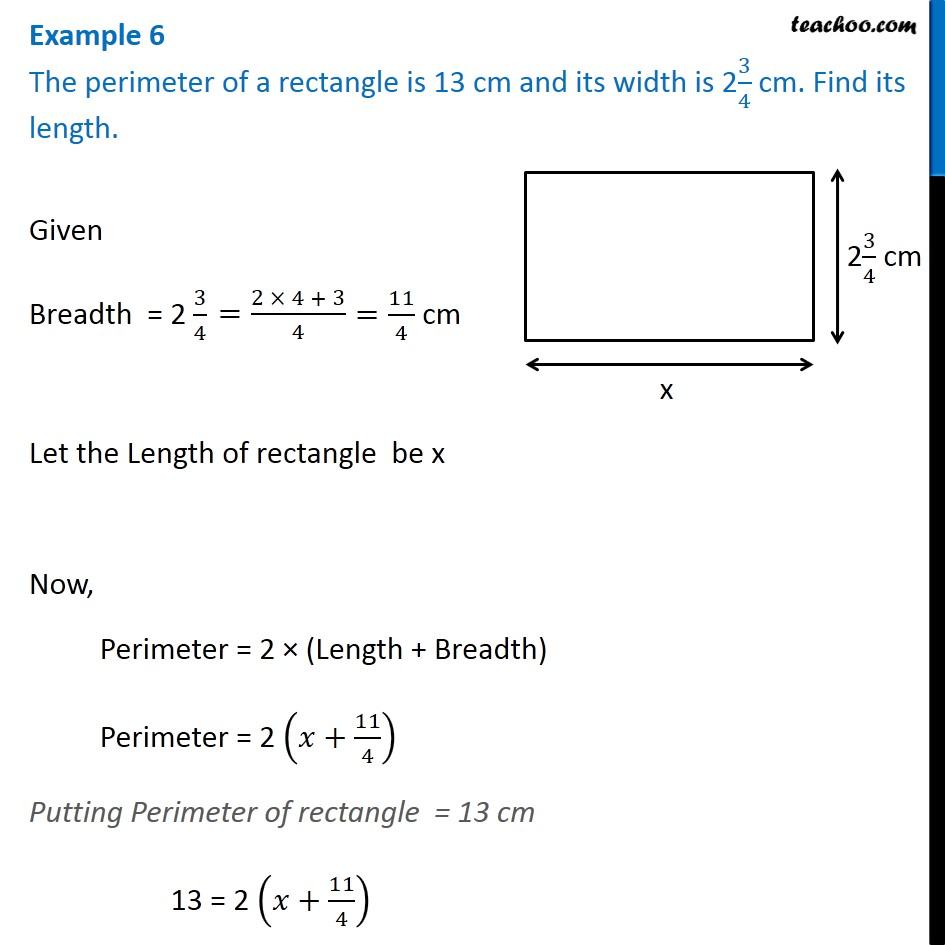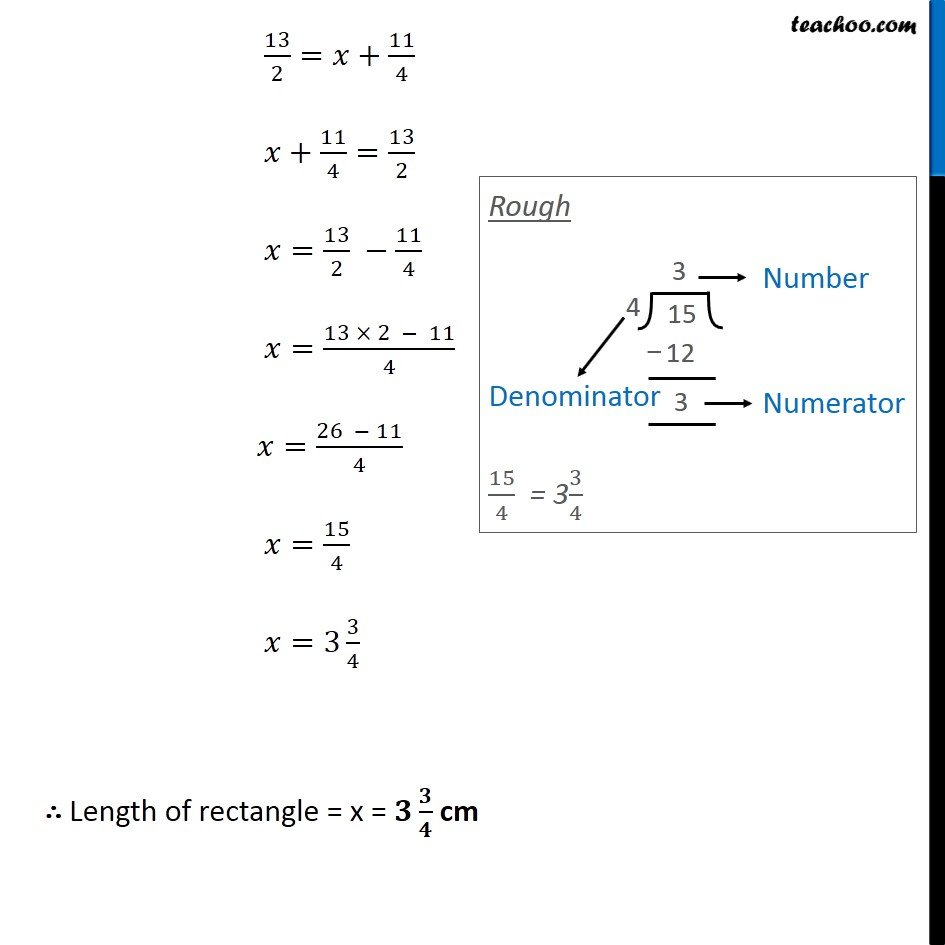1. Chapter 2 Class 8 Linear Equations in One Variable
2. Concept wise
3. Forming Linear Equations - Perimeter and Area

Transcript

Example 6 The perimeter of a rectangle is 13 cm and its width is 23/4 cm. Find its length.Given Breadth = 2 3/4 Let the Length of rectangle be x Now, Perimeter = 2 (Length + Breadth) Perimeter = 2 ( +11/4) Putting Perimeter of rectangle = 13 cm 13 = 2 ( +11/4) 13/2= +11/4 +11/4=13/2 =13/2 11/4 =(13 2 11)/4 =(26 11)/4 =15/4 =3 3/4 Length of rectangle = x = / cm